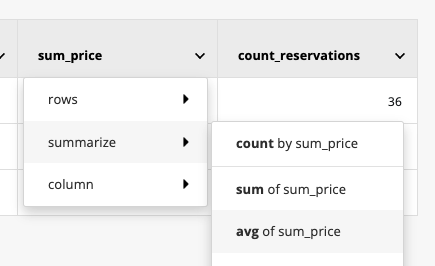# Get the average

## Average

The average function calculates the average of the values in a specific column.

Examples

To see the average price paid per reservation, you would calculate the average of the price column, not broken down by anything:To see the average price paid for reservations of each the different car brands, you would calculate the average of the price column broken down by the make column. The result would be:

0 out of 0 found this helpful

Have more questions? Submit a request

<% if (previousArticle || nextArticle) { %>
<% if (previousArticle) { %>
<% if (previousTitle) { %>

#### <%= previousTitle %>

<% } %> <%= previousArticle.title %>
<% } %> <% if (nextArticle) { %>
<% if (nextTitle) { %>

#### <%= nextTitle %>

<% } %> <%= nextArticle.title %>
<% } %>
<% } %>
<% if (items.length > 1) { %>